# Birch Clustering

## Introduction

The Birch clustering method is designed for clustering a large amount of numerical data. It is done by integrating hierarchical clustering and other clustering methods such as iterative partitioning. To overcome two difficulties of the agglomerative clustering method, we use

1)  Scalability

2)  The inability to undo what was done in the previous step.

## Clustering Feature And Clustering Feature Tree

Birch introduces two concepts for this;  clustering feature and the clustering feature tree used to summarise clustering representation. A clustering feature tree is a height-balanced tree that stores the clustering feature for hierarchical clustering. We need to know some cluster measures before we deep dive into the clustering feature and clustering tree.

measures of cluster

Given n  d-dimensional data object or point in a cluster then,

1) The centroid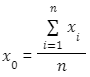Centroid is the middle of the cluster.

2) The radius R of the cluster is, R =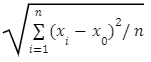The radius is the average distance from the member object to the cluster.

3) The diameter of the cluster, D =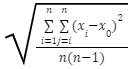The diameter of the cluster is the average pairwise distance within a collection.

Both radius and diameter reflect the tightness of the cluster around the centroid. Now we will move towards the clustering feature, i.e., CF.

Clustering feature is represented as, CF  = < n, LS, SS >

n =  Number of data points in the cluster

LS = The linear sum of n points

SS  = The squared sum of the data points.

For example, suppose we are given points (3,4), (2,6), (4,5), (4,7), (3,8). The value of n is 5. LS is (3+2+4+4+3) = 16, LS is (4+6+5+7+8) = 30. Similarly, we can find out the squared sum of the points that comes out to be (54, 190).

Hence the clustering feature CF is ( 5, ( 16, 30 ), ( 54, 190 ) )

the clustering feature is done, we must focus on the clustering feature tree. A clustering feature tree is an incremental insertion of new points.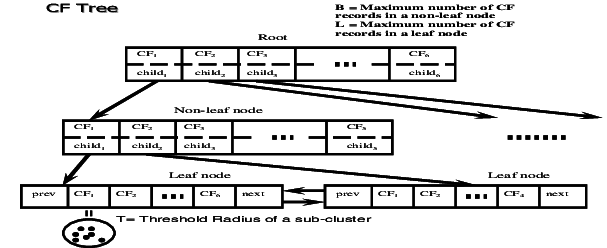source

A non-leafy node in a tree has a descendant or children. Leaf nodes store the sum of the clustering feature of their children and thus summarise clustering information about the children. The clustering feature has two parameters;  Branching factor(B) and Threshold(T).

The branching factor specifies the maximum number of children per non-leaf node.

Threshold specifies the maximum diameter of the sub-cluster stored at the leaf node of the tree.

Birch applies a multiphase clustering technique-

In Phase 1, it scans the database to build an initial Memory CF tree which can be viewed as a multilevel compression of the data that tries to preserve the inherent clustering structure of the data. It is just like B-tree insertion and deletion.

In Phase 2, Birch applies to cluster the leaf nodes of the CF tree.

The overall complexity of the algorithm is in order of n.

So to summarise, for each point in the input

• Find the closest leafy entry
• Add point to leaf entry and update clustering feature
• If the entry diameter is more remarkable than The maximum diameter, we split the leaf and possibly parents.

## Implementation

I will generate a dataset for clustering using Scikit learn's make_blobs() method. I will create 8 clusters with 600 randomly generated samples and plot the results in a scatter plot.

``````# Import required libraries and modules
import matplotlib.pyplot as plt
from sklearn.datasets.samples_generator import make_blobs
from sklearn.cluster import Birch

# Generating 600 samples using make_blobs
dataset, clusters = make_blobs(n_samples = 600, centers = 8, cluster_std = 0.75, random_state = 0)

# Creating the BIRCH clustering model
model = Birch(branching_factor = 50, n_clusters = None, threshold = 1.5)

# Fit the data (Training)
model.fit(dataset)

# Predict the same data
pred = model.predict(dataset)

# Creating a scatter plot
plt.scatter(dataset[:, 0], dataset[:, 1], c = pred, cmap = 'rainbow', alpha = 0.7, edgecolors = 'b')
plt.show()``````

Output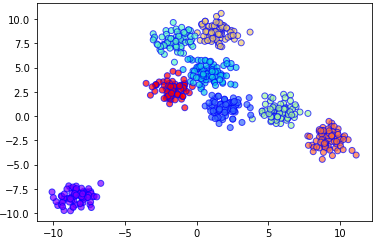## FAQs

1. What is the birch clustering method?

Ans. The Birch clustering method is designed for clustering a large amount of numerical data. It is done by integrating hierarchical clustering and other clustering methods such as iterative partitioning.

2. What does Branching factor in the clustering feature specify?

Ans. Branching factor in the clustering feature limits the maximum number of children per non-leaf node.

3. What does the Threshold in the clustering feature specify?

Ans. Threshold in clustering determines the maximum diameter of the sub-cluster stored at the leaf node of the tree.

4. What is a clustering feature tree?

Ans. Clustering feature tree is an incremental insertion of new points. A non-leafy node in a tree has a descendant or children. Leaf nodes store the sum of the clustering features of their children.

5. What is the significance of the radius and the cluster's diameter?

Ans. Both radius and diameter reflect the tightness of the group around the centroid.

## Key Takeaways

In this blog, we learned-

• The Birch reduction algorithm
• The Implementation